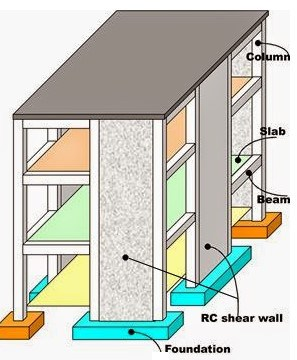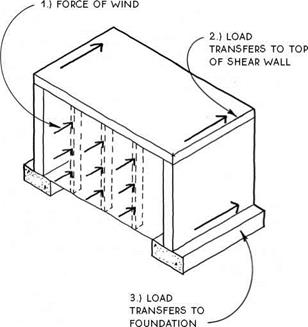## Shear Walls## Definition of Shear Wall:

Shear walls are the vertical structural element which resist the horizontal forces acting on a building structure. Shear wall can also be defined as a wall which are reinforced & made of braced panels to carry lateral forces. The thickness of the shear wall for regular buildings can be generally in the range of 150 mm to 400 mm.Shear Wall in a Building

Shear Wall in a Building

## Functions of Shear Wall:

The main functions of a Shear Wall can be described as follows :

• Providing Lateral Strength to building : Shear Wall must provided lateral shear strength to the building to resist the horizontal earthquake forces, wind forces and transfer these forces to the foundation.
• Providing Lateral Stiffness to building : Shear Walls provide large stiffness to building in the direction of their orientation, which reduces lateral sway of the building and thus reduces damage to structure.

Shear walls carry Horizontal Seismic forces downwards to the foundations. The overturning effects on shear walls are quite large. Thus, design of their foundations requires special foundations. If, the Shear wall is an exterior wall, then it will also carry the wind load & then it should also be design to resist the wind load and this load also get transfer to the foundation of the shear wall.

The various walls and co-existing frames in a building are linked at the different floor levels by means of the floor system, which distributes the lateral loads to these different systems appropriately. The interaction between the shear walls and the frames is structurally advantageous in that the walls restrain the frame deformations in the lower storey, while frame restrain the wall deformations in the upper storey. Frame-shear wall systems are generally considered in buildings up to about 40 storeys.Load Transfer mechanism in Shear Walls

Load Transfer mechanism in Shear Walls

The load attracted to each component is a function of position and relative stiffness. Compared to cantilever shear walls, stiff frames and short shear walls when subjected to horizontal load deform with a pure shear behavior, with a concave shape in the upper region and convex in lower region. Whereas cantilever shear walls has a flexural behavior, the deflection is convex like a BMD of a cantilever beam, throughout the height of the wall.Deformation in building with shear wallv

Deformation in building with shear wall

#### Design Example of Shear Wall:

Data Given :

Height of Shear Wall = 3 m

Length of Shear Wall = 4 m

Thickness of Wall = 100 mm

Solution :

Since cross walls are provided, the wall is braced.

So, h/t = 3000 / 100 = 30 mm

l/t = 4000/100 = 40 mm

Assume , both ends fixed ( restrained against rotation & displacement )

L (eff.) = 0.75*lo , where , lo is least of length & height

= 0.75 *3 = 2.25 m

L (eff.) / t =2250/100 = 22.5 > 12

As per Indian Standard , Permissible value of L (eff.)/t = 45 > 22.5

e (min.) = t/20 or 20 mm = 5 or 20 mm

Therefore, moment due to accidental eccentricity of 20 mm is considered. Additional eccentricity due to slenderness,

=  /(2000 * t) = (2.25)^2 * (1000)^2 / ( 2000 * 100 ) = 25.31 mm

Total Eccentricity =  +  = 20 + 25.3 = 45.3 mm

Moment  =  * 45.3 = 27.2 * 10^6  N-mm

For the axial load & moment , RCC Wall is designed similar to a RCC Column subjected to axial load & uniaxial moment.

/ * b * t = 600 * 10^3 / (20 * 100 * 1000) = 0.3

/(  * b * t^2 ) = 0.136

From SP16 chart , for Reinf. along two sides, Fe415 steel, d’/D = 0.1, Referring Chart 32,

p /  = 0.07  = 0.07

p = 0.07 * 20 = 1.4 %

Area of Steel = 1.4 / 100 * 100 * 1000 = 1400 mm2

Provide 16 mm @ 120 mm c/c as vertical reinf. for compression.

Horizontal provide a nominal reinf. of 0.4% of c/s Ast = 0.4 * 100 * 1000 * 100 = 400 mm2

Provide 8 mm @ 120 mm c/c .

Since Vertical Reinforcement is less than 2 %, no horizontal links are required.